Support the Monkey! Tell All your Friends and TeachersHome MonkeyNotes Printable Notes Digital Library Study Guides Study Smart Parents Tips College Planning Test Prep Fun Zone Help / FAQ How to Cite New Title Request

2.4 Tables of Trigonometric Function

When solving problems using trigonometric functions, either the angle is given and the value of t-function must be found, or the value of the t-function is given and angle must be found.

These two processes are inverse of each other. Thus inverse notations are used to express an angel in terms of the values of t-functions. For instant cos a = 0.5 can be put in the form a = cos -1 (-0.5) or a = Arc cos (- 0.5). The two expressions are read as Alpha equals to 'Inverse cos of (-0.5) or Alpha equals to Arc cos (- 0.5).

Both these operations can be done either using a calculator or using a trigonometric table. It should clearly be noted that both calculator or a table gives only approximate answers. Even so we use equality sign (=) but more correctly the use of approximation sign ( » ) is welcomed. Approximate values of the functions of acute angles are given in Tables of Natural Trigonometric functions. We shall use a Trigonometric table giving values to four decimal places. As it is clear that Tables cannot list all angles. Therefore, approximation must be used to find values between those given in the table. This method is known as Linear interpolation.

Assumption : Differences in functional values are directly proportional to the Differences in measures of angle over a very small interval.

Caution : This is not a real truth ! Yet it gives a better answer than just going for nearest value in the table.

Illustration 1

Using linear interpolation Find sin (240 43'), given that sin (240 40') = 0.4173 and sin (240 50') = 0.4200

Solution

We have sin (240 50') = 0.4200
and sin (24 0 40')= 0.4173
Difference for 10'= 0.0027

Owing to the assumption made if x is the difference for required 3' we have a ratio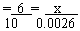\ x = 0.3 (0.0027) = 0.00081 » 0.0008 (rounded off to 4 decimal places)
Thus sin (240 40') = sin (24040') + sin (0.3') angle increase with an increment in its sine of angle and vice versa.
Thus sin (240 43') = 0.4173 + 0.0008 = 0. 4181Your browser does not support the IFRAME tag.

Illustration 2

Find cos (640 26'), given that cos (640 20') = 0.4331 and cos (640 30') = 0.4305

Solution

We have cos (640 30') = 0.4331
cos (640 20') = 0.4305

\ Tabular difference for 10' = 0.0026

\ The required difference for 6' =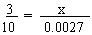(If x)

\ x = 0.6 (0.0026) = 0.00156 or 0.0016 (104 decimal places)

As the angle increases, the cosine of angle decreases. Thus cos (640 26') = 0.4331 - 0.0016

= 0.4315.

Illustration 3

Find tan (28.43)0, given that tan (28.40)0 = 0.5407 and tan (28.50)0 = 0.5430

Solution

=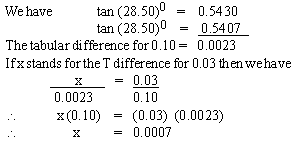As angle increases, the tangent of angle also increases.
Thus tan (28.43)0 = 0.5407 + 0.0007 = 0.5414

Illustration 4
Solve the right triangle in which a = 24.36 Ð A = 58053'.

Solution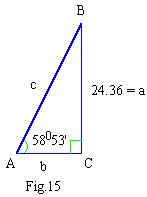In right triangle ABC
A + B + C = 1800
\ 58053' + B + C = 180 0, given that C = 900
\ B = 90 0 - 58053' = 310 7'
Using the formulas for t-ratios,
b / a = cot A, b = a cot A = 24.36 (0.6036) = 14.70 (\ cot A = 0.6036)
c/a = csc A, c = a csc A = 24.36 (1.1681) = 28.45
a/c = sin A, c = a / sin A = 24.36 / 0.8562 = 28.45
b/c = cos A, b = c cos A = 28.45 (0.5168) = 14.70

Note : To save time, consider Illustration 1
Step (1) sin (240 41') = 0.4173, take only 4173
(2) Find mentally the tabular difference 27 between 4200 (for sin 240 40') and 4173 (for sin 240 40')
(3) Difference for 3' = 0.3 (27) = 8.1 (rounded off).
(4) Add (since sine) to 4178 to get 4181 then sin 240 31' = 0.4181.

Illustration 5
Find angle A, given that sin A = 0.4234

Solution
We will not find this entry in the table.
However 0.4226 = sin 250 0'
0.4253 = sin 250 10'
Tabular difference = 0.0027
Now 0.4226 = sin 25.0'
0.4234 = sin A
0.0008 = partial difference
correction =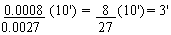(nearest minute)
Adding (since sine) the correction is A = 250 0' + 3' = 250 3'

Illustration 6
Find A, given that cot A = 0.6345

Solution
We have 0.6330 = cot 570 40' (from the table)
0.6371 = cot 570 30'
Tabular diff. = 0.0041
Now 0.6330 = cot 570 40'
0.6345 = cot A
Partial diff. = 0.0015
Correction =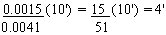(nearest minute)
subtracting (since cot), the correction is A = 570 40' - 4' = 570 36'.

Note : Saving the time as - (Consider Illustration 5)
Step (1) Locate the next smaller entry, 0.4226 = sin 250 0'. Use 4226 only.
(2) Find tabular diff. (mentally), 27.
(3) Find partial diff. (mentally), 8 between 4226 and 4234.
(4) Find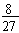(10') = 3' and add (since sine) to get A = 250 3'
Commit to memory the values of t-functions of angles measuring 00, 300 , 450, 600 and 90 0as follows:

(1) Write angle (q) in the given order in the 1st column and the t-ratios, sin q, cos q, tan q, csc q, sec q, cot q in the 1st row.
(2) Put 0, 1, 2, 3, 4 in sin q column (see the table), then put 4, 3, 2, 1, 0 in cos q column (see the table).
(3) Divide by 4 to each entry then find square root of each entry. These are values of sine and cosine ratios of angles 00, 300, 450, 60 0and 900
(4) Use tan q =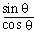q and reciprocal relations for csc q, sec q and cot q.

Thus we have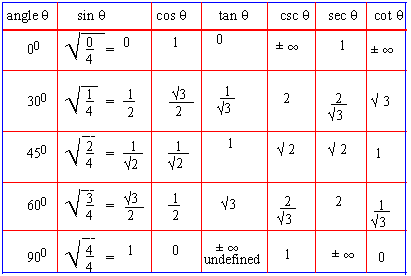Note that
1. As the measure of the angle increases values of sine ratios increases till 900 (p / 2 rad) and then decreases.
2. As the measure of the angle increases values of cosine ratios decreases till 900 (p / 2 rad) and then increases.
3. As the measure of the angle increases values of tangent ratio increases indefinitely till 900 (p / 2 rad), then decreases indefinitely.

Also note that

1. Since sin2q + cos2q =1 we have | sin q | £ 1 i.e. - 1 £ sin q £ 1 and |cos q | £ 1 i.e. - 1 £ cos q £ 1 for all q . These are called bounds of sine and cosine ratios.
2. Since csc q =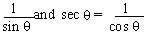we have,
csc q ³ 1 or csc q £ 1 and sec q ³ 1 or sec q £ 1 (numerically) for admissible values of q .
3. tan q and cot q can take all real values of q .

Index

2.1 Trigonometric Ratio of Acute Angles
2.2 Fundamental Relation between the trigonometric Ratios of an angle
2.3 Functions of General Angles or t Ratio
2.4 Tables of Trigonometric Function

Supplementary Problems

Chapter 3Search: All Products Books Popular Music Classical Music Video DVD Toys & Games Electronics Software Tools & Hardware Outdoor Living Kitchen & Housewares Camera & Photo Cell Phones Keywords: